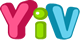0
Are you sure you want to quit the game?
How to play:
1. The objective is to fill the grid in with the digits 1 to 3 (depends on the grid size)
2. Each row contains exactly one of each digit
3. Each column contains exactly one of each digit
4. Each bold-outlined group of cells is a cage containing digits which achieve the specified result using the specified mathematical operation: addition (+), subtraction (−), multiplication (×), and division (÷).
00:00